﻿ Estimation of Import Demand Function Using ARDL Method: Evidence from NepalPublications are Open
Access in this journal
Article Versions
Export Article
• Normal Style
• MLA Style
• APA Style
• Chicago Style
Research Article
Open Access Peer-reviewed

### Estimation of Import Demand Function Using ARDL Method: Evidence from Nepal

Kamal Raj Dhungel
International Journal of Econometrics and Financial Management. 2018, 6(2), 45-52. DOI: 10.12691/ijefm-6-2-3
Received November 08, 2018; Revised December 18, 2018; Accepted December 29, 2018

### Abstract

Nepal’s dependence on remittance has been increasing over the years. This has the direct positive impact on overall consumption and in turn indirect positive impact on import. This paper aims to estimate the import demand function during the period 1990-2017 with the help of autoregressive distributed lag (ARDL) model. Bound testing conforms the existence of cointegration among the variables (import, remittance, gross domestic product, and consumer price index). The elasticity coefficient of import with respect to remittance is found 1.37 and 0.97 in the short and long run respectively. It implies that a 1% increase in remittance leads to increase the import by 1.37% in the short run and 0.97% in the long run respectively. Similarly, the elasticity coefficient of import with respect to GDP is found 0.24 and 0.14 in the short and long run respectively. It implies that a 1% increase in GDP leads to increase the import by 0.24% in the short run and 0.14% in the long run respectively. The elasticity coefficient of import with respect to CPI is found insignificant. These findings proved that the remittance is the key factor to increase the import in Nepal.

### 1. Background

Since 1990, openness has been followed by deindustrialization and migration in Nepal. Deindustrialization weakens domestic economy. Newer jobs have not been created. Obviously, unemployment rises. Deindustrialization limits new employment opportunities to solve the burning problem of unemployment. It necessitates sending people where their labor is demanded. Migration to foreign land is becoming the major alternative to addressing the problem of unemployment. Policies are being placed accordingly to encourage migration. Responses to these policies are positive, resulting a flock of Nepalese youths, accounted over a scale of 1500 a day flying to foreign countries. No matter the amount they are earning from their work, a major portion of earning is being sent back home to support the livelihood of their family. This has gradually helped to shift the base of the economy from mere subsistence farming to remittance. An increase in household income increases the purchasing power of the people, particularly the poor people of bottom two quintiles. As household income of migrant families increases due to receipt of remittance, so they may have propensity to consume more which will increase the demand of goods Mukit et al. (2013) 1. Households with remittance are supposed to consume more than what they consume without remittance. Remittance increases their demand of goods and services.

Nepal’s enormous development potentiality almost remains idle and is waiting for right strategy and proper and deterministic policies in action. Lacking deterministic policies if any were not in effect on the one and capital inadequacy on the other make things more complex to assume to exploit development potentiality. Tourism, hydropower, Himalayan herbs and agriculture itself are the potential sectors to develop. These sectors if developed will produce gold to Nepalese people which in turn create numerous job opportunities. For many years this has been becoming a day dream, and Nepalese are migrating to the extent of nearly four million in search of survival. They obviously are sending a part of their income earned in foreign land, constitute US\$6.9 billion in 2017 accounted 28% of GDP in their home to cover their family expenses. Total income from remittance was four times greater than earning from exports constitute 10% of GDP, 49 times greater than FDI accounted 0.8% of GDP, 19 times greater than the earning from tourism accounted 10% of GDP and nine times greater than aid accounted 15% of GDP in 2017. Tourism is a promising sector in Nepal however, it does not ye treceive proper development action. This indicates that the progress of domestic sector that earn foreign exchange is not as encouraging as remittance. This, of course, has a significant impact on Nepal’s economy. It definitely increases the household income and thereby increases the purchasing power of the mass of people in general and poor people at the bottom of the economic hierarchy. Remittance fuels their propensity of migrant families to consume more, and thereby increase the demand of goods. These households consume more than what they would have without remittance. It increases the demand of goods and services. As deindustrialization limits the ability to produce goods domestically, it forces the national economy to depend on imports to meet the increased demand. This has been increased the volume of imports over the years. Out of this nearly 80% has been used in consumption. It implies that households with remittance, naturally, have tendency to increase their level of consumption. The consequence is that more goods and services are demanded in the economy. Two strategies are available for this to follow-increase either domestic production or import. Domestic production is limited. Obviously, import, constitutes US\$ 119 billion accounted 42% of GDP in 2017 has become a means to meet an end. In this context, remittances help to correct balance of payment which in turn reduce current account balance. Since 1990s planners, policy makers and development experts in Nepal have focused on the role of remittances in economic development. Adenutsti & Ahortor (2008) 2 have opined that remittances cover scarce domestic resources, inadequate private capital inflow, and scarce official development assistance (ODA) in developing countries. As described in above paragraphs, remittance does not help to produce goods domestically to supply to meet the growing demand of people. This of course has made the economy to depend on import, which has been increasing over the years.

### 2. Trend of Remittance and Import

Both remittance and import have been increasing over the years. During 2009-2017, the inflow of remittance has been increasing. In 2009, the total amount of remittance was US\$3 billion which jumped to US\$6.9 billion in 2017 increased alarmingly at an annual growth rate of 110%. The share of remittance to GDP in 2009 was 23.2%. It reached 28.3% in 2017 increased at an annual growth rate of 22% (Table 1). A simple logic is that increased inflow of remittance would increase the level of consumption as government study has proved that nearly 80% of remittance was spent on consumption. Increase in consumption obviously increases the demand for goods and services that can be fulfilled either through imports or by expanding domestic production. In lack of the latter, Nepal’s import is the means to deliver the goods in the market. In 2009, Nepal imported goods to the tune of US\$5.1 billion nearly double the amount of remittance which jumped to US\$11.6 billion in 2017, increased at the annual growth rate of 127%. The share of import to GDP was 34.7% in 2009 which reached 42% in 2017 increased at the annual growth rate of 21%. However, during the same period there was negative growth in exports. In 2009, Nepal exported goods to the tune of US\$1.6 billion, less than three times of import, It reached US\$2.4 billion in 2017, five times lesser than the import, increased at the annual growth rate of 50%. Declining rate of export and the increasing rate of import produces trade imbalance thereby making the balance of payment unfavourable. The above facts prove that import and remittance move in the same direction. The co-movement of these variables is given in Figure 1, which shows that both of these variables have non-stationary increasing tendency.

• Figure 1. Trend of import and remittance

### 3. Review of Literature

Various applied research studies [(Nazif & Jaehyuk (2015) 3, Big Ben (2016) 4, Khurram and Syed (2012) 5, Abdulsalam (2015) 6, Al-Khulaifi (2013) 7, Aldakhil & Nourah (2002) 8, Zhou & Dube (2011) 9, Emmanuel & Mooya (2013) 10, Sulaiman & Saba (2016) 11] were undertaken in developed as well developing countries. These studies includes a number of economic and noneconomic variables such as relative prices, real effective exchange rate, final consumption, FDI, foreign reserves, exports, remittance and financial development among other macroeconomic variables. However, considering the scope of present study following section emphasis is given to relevant studies related to impact of remittance on import. Using bound test Tang and Nair (2002) 12 analyzed the stability of import demand function in Malaysia. They found cointegration between import, income and relative price. Their study estimates income and relative price elasticities of 1.5 per cent and -1.3 per cent, respectively. Ahmed et al. (2014) 13 examined the short-run and long-run relationships between imported goods and workers’ remittances in Pakistan over the period 2008–2012 employing Johansen cointegration and Granger causality. The finding showed a positive and significant impact of remittances on imports. However, Granger causality indicated a unidirectional causality runs from imports to remittances. Bashier (2018) 14 applied the autoregressive distributed lagged (ARDL) model to estimate the import function in Jordon over the period 1975–2016. The finding of this study is that the bounds testing provided evidence of the existence of a long-run equilibrium relationship between the included variables. Using the OLS method, Munir et al. (2007) 15 estimated the import function for Pakistan. The finding showed that remittances and GDP have positive and significant impacts on imports, while real effective exchange rate was negative. M. Sayed (2014) 16 employed vector error correction (VECM) to investigate the causality between import and remittance using annual data over the period 1991–2011 for Egypt. The finding reveals the unidirectional causality running from remittances to imports implies that positive and significant impact of remittance on imports. Using vector error correction model (VECM) to monthly data over the period 2001-2011 Bhatta (2013) 17 examined the impact of remittance on import. The finding shows that remittances have positive impact on imported merchandised goods and services with unidirectional causality runs from remittances to imports, Khair and Nazakat (2005) 18 applied the OLS method using quarterly data over the period 1975–2004 in Pakistan. The findings indicated that remittances have a positive and significant impact on imports. Dhungel (2014a) 19 and Dhungel(2016) 20 undertook a couple of studies covering the periods (1974-12 & 13) to investigate the short and long run causality between gross domestic product and remittance using vector error correction model (VECM). This study finds that the unidirectional causality running from the 1) remittance to gross domestic product in the short run, 2) Gross domestic product to remittance in both short and long run and 3) remittances to economic growth in both short and long run. Dhungel (2015) 21 has estimated marginal propensity to import. From empirical analysis it appears that the import and remittance variables are positively correlated over the time period of 1974 to 2012. The marginal propensity to import during that time period is found 0.48. It indicates that a 1 percent change in remittance will change the import by 0.48 percent. It indicates that additional increase in remittance by say rupees 100 in Nepali economy will increase the import by 48 rupees. The implication is that nearly 50 percent of the remittance that Nepali youths are supposed to have sent home from abroad countries has been spent in importing goods. Dhungel (2014b) 22 to investigate the short and long run equilibrium between the electricity consumption and foreign aid of Nepalese economy during 1974-2012. He has conducted Unit root test, co-integration test and finally error correction model are the econometric tools to establish the relationship between electricity consumption and foreign aid. He found short and long run relationship between electricity consumption and foreign aid. The long run elasticity coefficient reveals that the 1% change in foreign aid will change the electricity consumption by 0.46%. The results of ECM indicate that there is both short and long run equilibrium in the system. The coefficient of one period lag residual is negative and significant which represent the long run equilibrium. The coefficient is -0.336 meaning that system corrects its previous period disequilibrium at a speed of 33.6% annually to reach at the steady state.

### 4. Data and Model

4.1. The Data and Their Characteristics

This study incorporates four variables- Import (IMP) in million US\$ as dependent variable, gross domestic product (GDP) in million US\$, remittance (REM) in million US\$ and consumer price index (CPI) as independent variables to examine the impact of remittance on import. The data of all the variables are collected from the World Bank web site. The data (series) of variables under consideration are transform in natural logarithm except CPI.

4.2. Feature of Variables

(Table 2) represents the summary of the characteristics of variables. The sample size is 28 covering the period 1990-2017 The mean value of import, remittance, GDP and CPI respectively are 7.9, 5.8, 6.3 and 53.9 with standard deviation 0.8, 0.5, 2.0 and 31.1. Standard deviation indicates the scatter of data around the mean value. Similarly, the value of Jarque-Bera, a measure of the normality of series is 3.2, 3.4, 3.3 and 2.1 with probability 0.2, 0.2, 0.2 and 0.3 respectively. It implies that the series under consideration are normally distributed.

4.3. Graphical Representation of Data

(Figure 2) displays the insight view of data at their level. Data series of all the variables imp, REM. GDP and CPI are non-stationary. In such a case, data are to be tested by applying specific standard tests to make them stationay. If such data used to estimate the relationship between the variables would produce misleading results. Therefore, it is essential to convert them into stationary. One way of making them stationary is to convert them into first difference. (Figure 3) displays the insight view of stationary series in their first difference of all the variables under consideration.

• Figure 2. Graphical representation of data on their level form
• Figure 3. Graphical representation of data on first difference
4.4. Model Specification

To establish the relationship between import and remittance the following model specification is used.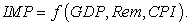(1)

The simple linear functional form of model (1) is as follows.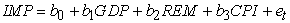(2)

where, IMP= import, GDP = gross domestic product, REM = remittance (in million US\$ expressed in natural logarithm) and CPI = consumer price index.

Cointegration

Several methods are available for investigating the cointegration among which pesaran, et al (2001) 23 developed ARDL bounds testing approach to cointegration. This approach is applicable irrespective of the order of integration such as I(0) or I(1). The unrestricted error correction method used to examine the short and long run relationship as specified in model (3). In case the calculated F-statistics exceeds the upper critical bound, then the series are cointegrated, and if it is below the lower critical bound there is no cointegration. If the calculated F-statistics is between the UCB and the LCB, then decision about cointegration is inconclusive. The critical bounds are taken from Pesaran and Pesaran (1997) 24.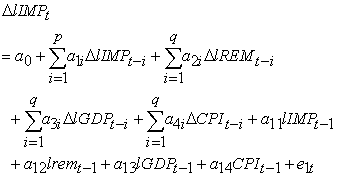(3)

where, Δ = Difference operator, l is natural logarithm, a0 is the constant,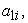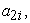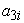and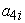(i=0,1,2…n) are the short run coefficient and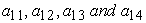are the long run coefficients. p (= 2) is the optimum lag of dependent variable, q (= 4) is the optimum lag of independent variables, IMP, REM, GDP and CPI are the import, remittance gross domestic product and consumer price index respectively. The optimum lag length is selected based on the Akaike information criteria.

To establish relationship between the variable, model (3) is used to bound test of cointegration.

1) If there is no cointegration the ARDL (p, q) model is specified as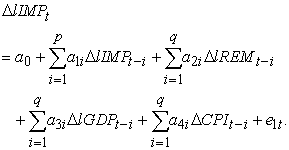(4)

Equation (4) is a short run model which is to be estimated only when there is no cointegration.

2) If there exists cointegration, the error correction model (ECM) is specified as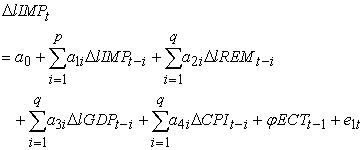(5)

where, ψ is the speed of adjustment parameter with a negative sign and ECT is the error correction term. a1i, a2i, a3i and a4i are the short run dynamic coefficients of the model’s adjustment long run equilibrium.

### 5. Empirical Findings

5.1. Unit Root Test

The present study attempts to assess impact of remittance on import and economic growth with the help of ARDL model. The first step in the ARDL model to apply is to determine the order of the integration. The order of integration should have I(0) or I(1) or the mixture of both. It is the prerequisite for the propose model to apply. This implies that the integration order of the included variables should not be I(2). Augmented Dickey Fuller (ADF) and Philips-Parron (PP) test have proved that all the variables included in the study are I(1) (Table 3). From both the test ADF and PP it is found that all the variables (IMP, REM, GDP and CPI) in first difference are I(1). None of them are I(2). Therefore, it allows us to apply ARDL model to investigate the impact of remittance and trade on economic growth.

5.2. Bound Testing

Bound test for cointegration through ARDL approach is estimated using equation (3). It involves the comparison of the F-statistics against the critical values. The calculated F-statistics are reported in Table 4 with the corresponding critical values designed by Pesaran (2001) 23 and Narayan (2005) 25. The computed value of f-statistics is 5.05. This value is higher than the upper bound critical value at 5% significance level. According to the rule of hypothesis testing if the calculated value of test statistics is greater than the critical value at specified significance level we can reject the null hypothesis. Accordingly, calculated F value = 5.05 is higher than the upper bound critical vale of 3.06(Pesaran) and 4.22(Narayan) at 5% significance level. It rejects the null hypothesis of no cointegration among the variables import, remittance, GDP and CPI. It conforms that these variables have long run relationship or they are cointegrated.

5.3. Long Run Estimation

Having the variables under consideration cointegrated the next task is to estimate the long run model. Equation (2) is the long run model. The long run estimation results through ARDL (2,0,3,4) model is reported in (Table 5). The value of R-square is 0.96. It implies that 96 percent variation in import has been explained by remittance, GDP and CPI. The F-statistics (271.2) with probability (0.0) shows that the model is statistically significant. The estimated results are robust as there is no serial correlation as evidenced by LM test (9.22) with probability (0.1693).

The central or focus variable in this study is remittance. It is assumed that an increase in remittance inflow in Nepalese economy leads to proportionate increase in import is the pre-assumption of this study. Estimated results reveals that there is a positive impact of remittance on import. As expected the elasticity of import with respect to remittance is nearly proportional. The elasticity coefficient is 0.97. It indicates that proportionate change in remittance leads to nearly proportionate change in import. A 1% increase in remittance leads to an increase in imports by 0.97%. The elasticity of import with respect to GDP is inelastic. A 1% increase in GDP leads to 0.14% increase in import. Similarly, a one unit increase in CPI leads to 0.08 units increase in import.

5.4. Structural Break Test

The stability tests have been used to investigate the stability of long run and short run parameters. In doing so, cumulative sum (CUSUM) and cumulative sum of squares (CUSUMsq) tests have been employed as suggested by Pesaran and Shin (1999) 26, Brown et al. (1975) 27 and Pearson et al. (2001) 23. The graph of both CUSUM and CUSUMsq are presented in Figure 4 and Figure 5 respectively. The plots of both figures are between critical boundaries at 5% level of significance. It means they are within the lower and upper critical limits. It conforms the accuracy of short and long run parameters and verifies the stability of ARDL model for structural break. The findings of the estimated model are reliable and efficient.

• Figure 4. Plot of Cumulative Sum of Recursive Residuals
• Figure 5. Plot of Cumulative Sum of Squares of Recursive Residuals
5.5. Short Run Elasticities

Table 6 reports the results of short run elasticities. The error correction term represents the speed of adjustment towards equilibrium. It is negative and statistically significant as expected. It measures the speed at which import demand adjust to changes in the explanatory variables converging to its equilibrium level. Its coefficient is -(1.41). It implies that the speed of adjustment to equilibrium at the rate of 1.41% after shock. The disequilibrium of the previous year’s shock converges to import back to the long run equilibrium in the current year at the speed of 1.41% in Nepal. The short run impact of remittance to import is found elastic whereas the same for GDP and CPI is found inelastic. A 1% increase in remittance leads to 1.37% in import. It indicates that the proportionate change in import is larger than the proportionate change in remittance. The short run elasticity coefficient of imports as in the long run with respect to GDP is inelastic. A 1% increase in GDP leads to 0.24% in import. It indicates that the proportionate change in import is lesser than the proportionate change in GDP. The impact of CPI on import seems insignificant.

### 6. Conclusion

The trade deficit in Nepal is mounting in recent years. This is the outcome of increased consumption in both the government and private sector as well as the depressing export. The export and import as percent of GDP in 2017 was 9.6 and 42 respectively. Increased consumption expenditure of both public and private sector has been largely financed by remittance. The consumption of goods (necessary, comfort and luxury) has been delivered through import. Thus, remittance helps to i) increase consumption expenditure directly and ii) increase import indirectly. In this light, present study aims to estimate the import demand function with respect to remittance, gross domestic product and consumer price index using ARDL approach. The order of these variables I(1) investigated through unit root test. Bound test proves that there exists long run relationship between the variables. The elasticity coefficient of import with respect to remittance is found 1.37 and 0.97 in the short and long run respectively. It implies that a 1% increase in remittance leads to increase the import by 1.37% in the short run and 0.97% in the long run respectively. Similarly, the elasticity coefficient of import with respect to GDP is found 0.24 and 0.14 in the short and long run respectively. It implies that a 1% increase in GDP leads to increase the import by 0.24% in the short run and 0.14% in the long run respectively. The elasticity coefficient of import with respect to CPI is found insignificant. Among other thing, remittances contribute to raise import as Nepal is unable to produce goods domestically to meet the growing demand.

### ReferencesThis work is licensed under a Creative Commons Attribution 4.0 International License. To view a copy of this license, visit http://creativecommons.org/licenses/by/4.0/